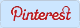Probability Worksheets - Coins, Cards, Dice, Spinner and more

 Home Number Sense Pre-Algebra Algebra Geometry Statistics Math Test Membership LoginMath Worksheets > Statistics Worksheets > Probability

Probability Worksheets

 Free printable probability worksheets for kids from grade 1 to grade 3 include basic probability based on more likely, less likely, equally likely, certain and impossible events. Worksheets based on identifying suitable events and simple spinner problems also included. Probability worksheets for kids from grade 4 and up include probability on single coin, two coins, days in a week, months in a year, fair die, pair of dice, deck of cards, numbers and more. Mutually exclusive and inclusive events, probability on odds and other challenging probability worksheets are useful for grade 6 and up students. Download All Probability Worksheets (1.42 MB) Members, Please LOG IN to download. Not a Member? Please SIGN UP to gain complete access.

Probability on Coins:

Simple probability worksheets based on tossing single coin or two coins. Identify the proper sample space before finding probability.

 Probability in a single coin toss Probability in pair of coin -1 Probability in pair of coin-2

Probability on Days and Months:

Fun filled worksheets based on days in a week and months in a year. Sample space is easy to find but care is required in identifying like events.

 Days of a week Months of a year-1 Months of a year-2

Probability on Odds:

Probability on odds worksheets can be broadly classifieds as favorable to the events or against the events.

 Odds worksheet-1 Odds worksheet-2 Odds worksheet-3

Probability on Fair Die:

Fair die is numbered from 1 to 6. Understand the multiples, divisors and factors and apply it on these probability worksheets.

 Simple numbers Multiples and divisors Factors Mutually exclusive and inclusive

Probability on Pair of Dice:

Sample space is little large which contains 36 elements. Write all of them in papers before start answering on probability questions.

 Based on numbers Based on sum and difference Based on multiples and divisors Based on factors Mutually exclusive and inclusive

Probability on Numbers:

Students should learn the concepts of multiples, divisors and factors before start practicing these worksheets.

 Probability on numbers -1 Probability on numbers - 2 Probability on numbers - 3 Probability on numbers - 4 Probability on numbers - 5

Probability on Deck of Cards:

Deck of cards contain 52 cards, 26 are black, 26 are red, four different flowers, each flower contain 13 cards such as A, 1, 2, ..., 10, J, Q, K

 Deck of cards-worksheet 1 Deck of cards-worksheet 2 Deck of cards-worksheet 3

Probability on Independent and Dependent:

Challenging probability worksheets for kids based on dependent and independent events with various real life applications.

 Based on deck of cards Based on marbles Based on cards

Probability on Spinners:

Interactive worksheets for lower grade kids to understand the probability using spinners. Colorful spinners are included for more fun.

 Spinner worksheets on numbers Spinner worksheets on colors

Probability on Different Events:

Basic probability worksheets for beginners to understand the different type of events such as more likely, less likely, equally likely and so on.

 Balls in container Identify suitable events Mutually inclusive and exclusive events
 Free Math Test Practice